Assertion & Reason Test: Polynomials - 1

# Assertion & Reason Test: Polynomials - 1 - Class 10

Test Description

## 10 Questions MCQ Test Mathematics (Maths) Class 10 - Assertion & Reason Test: Polynomials - 1

Assertion & Reason Test: Polynomials - 1 for Class 10 2023 is part of Mathematics (Maths) Class 10 preparation. The Assertion & Reason Test: Polynomials - 1 questions and answers have been prepared according to the Class 10 exam syllabus.The Assertion & Reason Test: Polynomials - 1 MCQs are made for Class 10 2023 Exam. Find important definitions, questions, notes, meanings, examples, exercises, MCQs and online tests for Assertion & Reason Test: Polynomials - 1 below.
Solutions of Assertion & Reason Test: Polynomials - 1 questions in English are available as part of our Mathematics (Maths) Class 10 for Class 10 & Assertion & Reason Test: Polynomials - 1 solutions in Hindi for Mathematics (Maths) Class 10 course. Download more important topics, notes, lectures and mock test series for Class 10 Exam by signing up for free. Attempt Assertion & Reason Test: Polynomials - 1 | 10 questions in 20 minutes | Mock test for Class 10 preparation | Free important questions MCQ to study Mathematics (Maths) Class 10 for Class 10 Exam | Download free PDF with solutions
 1 Crore+ students have signed up on EduRev. Have you?
Assertion & Reason Test: Polynomials - 1 - Question 1

### Direction: In the following questions, a statement of assertion (A) is followed by a statement of reason (R). Mark the correct choice as:Assertion : If α,β, γ are the zeroes of x3 - 2x2 + qx - r and α + β = 0 , then 2q = r.Reason : If α,β, γ are the zeroes of ax3 + bx2 + cx + d , then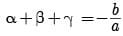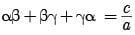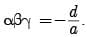Detailed Solution for Assertion & Reason Test: Polynomials - 1 - Question 1

Clearly, Reason is true. [Standard Result]

α + β + γ = -(- 2) = 2

0 + γ = 2

γ = 2

αβγ = -(- r) = r

αβ(2) = r

αβ = r/2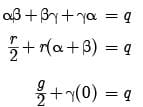γ = 2q Assertion is true.

Since, Reason gives Assertion.

Assertion & Reason Test: Polynomials - 1 - Question 2

### Direction: In the following questions, a statement of assertion (A) is followed by a statement of reason (R). Mark the correct choice as:Assertion : P(x) = 4x3 – x2 + 5x4 + 3x – 2 is a polynomial of degree 3.Reason : The highest power of x in the polynomial P(x) is the degree of the polynomial.

Detailed Solution for Assertion & Reason Test: Polynomials - 1 - Question 2
The highest power of x in the polynomial P(x) = 4x3 – x2 + 5x4 + 3x – 2 is 4.

Therefore, the degree of the polynomial P(x) is 4.

Assertion & Reason Test: Polynomials - 1 - Question 3

### Direction: In the following questions, a statement of assertion (A) is followed by a statement of reason (R). Mark the correct choice as:Assertion (A): If the zeroes of a quadratic polynomial ax2 + bx + c are both positive, then a, b and c all have the same sign.Reason (R): If two of the zeroes of a cubic polynomial are zero, then it does not have linear and constant terms.

Detailed Solution for Assertion & Reason Test: Polynomials - 1 - Question 3
Let α and β be the roots of the quadratic polynomial. If α and β are positive then α + β = -b/a it it shows that -b/a is negative but sum of two positive numbers (α, β) must be +ve i.e., either b or a must be negative. So, a, b and c will have different signs.

∴ Given statement is incorrect.

In case of reason:

Let β = 0, γ = 0

f(x) = (x – α) (x – β) (x – γ)

= (x – α) x · x

⇒ f(x) = x3 – αx2 which has no linear (coefficient of x) and constant terms.

∴ Given statement is correct.

Thus, assertion is incorrect but reason is correct.

Assertion & Reason Test: Polynomials - 1 - Question 4

Direction: In the following questions, a statement of assertion (A) is followed by a statement of reason (R). Mark the correct choice as:

Assertion : (2 - √3) is one zero of the quadratic polynomial then other zero will be (2 + √3).

Reason : Irrational zeros (roots) always occurs in pairs.

Detailed Solution for Assertion & Reason Test: Polynomials - 1 - Question 4
As irrational roots/zeros always occurs in pairs therefore, when one zero is (2 - √3) then other will be (2 + √3). So, both A and R are correct and R explains A.
Assertion & Reason Test: Polynomials - 1 - Question 5

Direction: In the following questions, a statement of assertion (A) is followed by a statement of reason (R). Mark the correct choice as:

Assertion : x3 + x has only one real zero.

Reason : A polynomial of nth degree must have n real zeroes.

Detailed Solution for Assertion & Reason Test: Polynomials - 1 - Question 5
Reason is false [a polynomial of nth degree has at most n zeroes.] Again, x3 + x = x(x2 + 1) which has only one real zero i.e. x = 0

[x2 + 1 ≠ 0 for all x ∈ R]

Assertion & Reason Test: Polynomials - 1 - Question 6

Direction: In the following questions, a statement of assertion (A) is followed by a statement of reason (R). Mark the correct choice as:

Assertion (A): The value of k for which the quadratic polynomial kx2 + x + k has equal zeroes are ± 1/2.

Reason (R): If all the three zeroes of a cubic polynomial x3 + ax2 – bx + c are positive, then at least one of a, b and c is non-negative.

Detailed Solution for Assertion & Reason Test: Polynomials - 1 - Question 6
In case of assertion

f(x) = kx2 + x + k (a = k, b = 1, c = k)

For equal roots b2 – 4ac = 0

⇒ (1)2 – 4(k) (k) = 0

⇒ 4k2 = 1

⇒ k2 = 1/4

⇒ k = ± 1/2

So, there are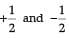values of k so that

the given equation has equal roots.

∴ Given statement is correct.

In case of reason:

All the zeroes of cubic polynomial are positive only when all the constants a, b, and c are negative.

∴ Given statement is incorrect.

Thus, assertion is correct but reason is incorrect.

Assertion & Reason Test: Polynomials - 1 - Question 7

Direction: In the following questions, a statement of assertion (A) is followed by a statement of reason (R). Mark the correct choice as:
Assertion : Zeroes of f(x) = x2 - 4x - 5 are 5, - 1
Reason : The polynomial whose zeroes are 2 + √3, 2 - √3 is x2 - 4x + 7.

Detailed Solution for Assertion & Reason Test: Polynomials - 1 - Question 7

Zeroes of f(x) = x2 − 4x − 5 are obtained by solving:
x2 − 4x − 5 = 0, which implies
x2 − 5x + x − 5 = 0 or, x(x − 5) + 1(x − 5) = 0
which means x = 5 or x = −1
Thus the assertion is correct.
However, the reason is incorrect.
The numbers given are not the zeroes  of x2 − 4x + 7

Assertion & Reason Test: Polynomials - 1 - Question 8

Direction: In the following questions, a statement of assertion (A) is followed by a statement of reason (R). Mark the correct choice as:

Assertion : If one zero of poly-nominal p(x) = (k2 + 4)x2 + 13x + 4k is reciprocal of other, then k = 2.

Reason : If (x – a) is a factor of p(x), then p(a) = 0 i.e. a is a zero of p(x).

Detailed Solution for Assertion & Reason Test: Polynomials - 1 - Question 8
Let α, 1/α be the zeroes of p(x) then we have

Product of Zeroes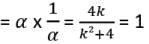⇒ k2 – 4k + 4 = 0

⇒ (k – 2)2 = 0 ⇒ k = 2

Assertion & Reason Test: Polynomials - 1 - Question 9

Direction: In the following questions, a statement of assertion (A) is followed by a statement of reason (R). Mark the correct choice as:

Assertion (A): The graph of y = p(x), where p(x) is a polynomial in variable x, is as follows: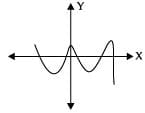The number of zeroes of p(x) is 5.

Reason (R): If the graph of a polynomial intersects the x-axis at exactly two points, it need not be a quadratic polynomial.

Detailed Solution for Assertion & Reason Test: Polynomials - 1 - Question 9
In case of assertion: Since the graph touches the x-axis 5 times, So, the number of zeroes of p(x) is 5.

∴ Given statement is correct.

In case of reason:

If a polynomial of degree more than two has two real zeroes and other zeroes are not real or are imaginary, and then graph of the polynomial will intersect at two points on x-axis.

∴ Given statement is correct:

Thus, both assertion and reason are correct but reason is not the correct explanation for assertion.

Assertion & Reason Test: Polynomials - 1 - Question 10

Direction: In the following questions, a statement of assertion (A) is followed by a statement of reason (R). Mark the correct choice as:

Assertion : x2 + 4x + 5 has two zeroes.

Reason : A quadratic polynomial can have at the most two zeroes.

Detailed Solution for Assertion & Reason Test: Polynomials - 1 - Question 10
Assertion: Consider the given polynomial x 2 + 4x + 5

Because the degree of the polynomial is 2. It is a quadratic polynomial. We know that the quadratic polynomial has at the most two zeroes.

∴ x2 + 4x + 5 has two zeroes.

∴ Assertion is true.

Reason: Clearly, Reason is true.

Since both the Assertion and Reason are true and Reason is a correct explanation of Assertion.

## Mathematics (Maths) Class 10

115 videos|478 docs|129 tests
Information about Assertion & Reason Test: Polynomials - 1 Page
In this test you can find the Exam questions for Assertion & Reason Test: Polynomials - 1 solved & explained in the simplest way possible. Besides giving Questions and answers for Assertion & Reason Test: Polynomials - 1, EduRev gives you an ample number of Online tests for practice

## Mathematics (Maths) Class 10

115 videos|478 docs|129 tests